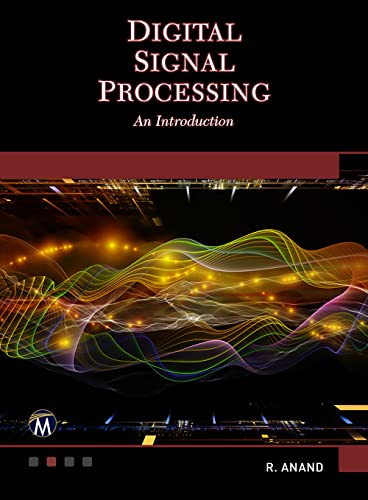# Digital Signal Processing: An Introduction

Description

Designed to cover the fundamental concepts of digital signal processing, the book introduces topics such as discrete-time signals, the z-transform, frequency analysis, discrete and fast Fourier transforms, digital filters, FIR, statistical DSP, applications, and more. DSP has been applied in most disciplines ranging from engineering to telecommunications, and from astronomy to medical imaging. This book focuses on the fundamentals of DSP, namely on the representation of signals by mathematical models and on the processing of signals by discrete-time systems.

FEATURES:

• Designed to cover the fundamental concepts of DSP
• Introduces topics such as discrete-time signals, the z-transform, frequency analysis, discrete and fast Fourier transforms, digital filters, FIR, statistical DSP, applications, and more
• Features a variety of exercises and a glossary